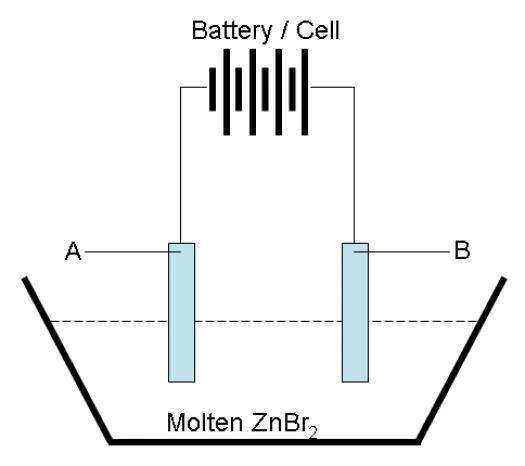# Electrolysis Of Molten Zinc Bromide

6 Questions | Total Attempts: 960SettingsIn this quiz on the process of electrolysis, a technique in the scientific field of chemistry wherein direct electric current is used to drive a chemical reaction, we’ll be taking a specific look at the

• 1.
Bromide ions would move towards electrode A
• A.

True

• B.

False

• 2.
Electrode A is known as the anode.
• A.

True

• B.

False

• 3.
The cathode half equation is _______________.(a) Br-(l) --> Br2(g) + e(b) 2Br-(l) --> Br2(l) + 2e(c) Zn2+(l) + 2e--> Zn(s)(d) Zn2+(l) + 2e --> Zn(l)
• A.

(a)

• B.

(b)

• C.

(c)

• D.

(d)

• 4.
The anode half equation is _______________.(a) 2Br-(l) --> Br2(g) + e(b) 2Br-(l) --> Br2(l) + 2e(c) Zn2+(l) + 2e--> Zn(s)(d) Zn2+(l) + 2e --> Zn(l)
• A.

(a)

• B.

(b)

• C.

(c)

• D.

(d)

• 5.
A reduction reaction has taken place at electrode _______________.
• A.

A

• B.

B

• 6.
The chloride ions are _______________ in the electrolysis reaction.
• A.

Reduced

• B.

Oxidized

Related TopicsBack to top